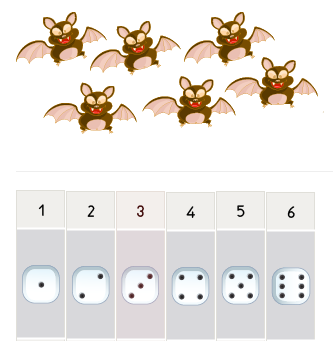# Counting numbers 1 to 6 - Counting and Number Recognition up to 6

## Learn how to count up to 6

Counting numbers 1 to 6 is a bold step to take in building a good math skill foundation for kids. Moreover, counting and number recognition up to 6 help kids to learn the numerical value and do simple interactive exercises that are designed to give a better understanding of number six.

Furthermore, as kids learn how to count up to 6, other math skills are being developed such as orderly counting from 1 to 6 and an understanding of the amount each numeral represents.

Another bold step is taken when kids learn how to count up to 6. This bold step is an introduction to the concept of addition, wherein given a round figure 5, a 1 is added to give the number 6. So kidos, as we are able to count from 1 to 5, we’ll just note that the next number after 5 is 6, thus 1, 2, 3, 4, 5, 6.• ## IN THE SAME CATEGORY

### Example- how many animals are there?Hey! kids, just rapidly glance down, and observe the quantity of dots on the dice and their corresponding numbers.

The dice below will enable you easily determine how many animals are there.

• Now, we begin by counting the animals. Let’s go…. 1, 2, 3, 4, 5, 6
• Secondly, we count the dotted dice with equal numbers as those of the animals

Hmmmmmm! Yes. Having counted all the dice, you’ll notice that the last dice has 6 spots on it, same number as the animals.

This makes it very easy because the number written above the 6 spotted dice is number 6.

Yeah! So there are 6 animals. Very good

•